Math Olympiad Test: Lines and Angles- 1

# Math Olympiad Test: Lines and Angles- 1

Test Description

## 10 Questions MCQ Test Mathematics Olympiad for Class 9 | Math Olympiad Test: Lines and Angles- 1

Math Olympiad Test: Lines and Angles- 1 for Class 9 2022 is part of Mathematics Olympiad for Class 9 preparation. The Math Olympiad Test: Lines and Angles- 1 questions and answers have been prepared according to the Class 9 exam syllabus.The Math Olympiad Test: Lines and Angles- 1 MCQs are made for Class 9 2022 Exam. Find important definitions, questions, notes, meanings, examples, exercises, MCQs and online tests for Math Olympiad Test: Lines and Angles- 1 below.
Solutions of Math Olympiad Test: Lines and Angles- 1 questions in English are available as part of our Mathematics Olympiad for Class 9 for Class 9 & Math Olympiad Test: Lines and Angles- 1 solutions in Hindi for Mathematics Olympiad for Class 9 course. Download more important topics, notes, lectures and mock test series for Class 9 Exam by signing up for free. Attempt Math Olympiad Test: Lines and Angles- 1 | 10 questions in 10 minutes | Mock test for Class 9 preparation | Free important questions MCQ to study Mathematics Olympiad for Class 9 for Class 9 Exam | Download free PDF with solutions
 1 Crore+ students have signed up on EduRev. Have you?
Math Olympiad Test: Lines and Angles- 1 - Question 1

### What is the measure of an angle which is equal to 5 times its supplement?

Detailed Solution for Math Olympiad Test: Lines and Angles- 1 - Question 1

Let the measure of angle be x°
∴ X° = 5(180° - x)
⇒ 6x = 5 × 180°
⇒ x = 150°

Math Olympiad Test: Lines and Angles- 1 - Question 2

### Two complementary angles are in the ratio 2 : 7. The measure of a smaller angle is:

Detailed Solution for Math Olympiad Test: Lines and Angles- 1 - Question 2

Let the measure of angles be 2x° and 7x° respectively
∴ 2x° + 7x° = 90°
⇒ 9x = 90°
⇒ x = 10°
∴ Measure of smaller angle
= 2x = 2 × 10° = 20°

Math Olympiad Test: Lines and Angles- 1 - Question 3

### OP bisects ∠BOC and OQ, ∠AOC. Find the measure of ∠POQ.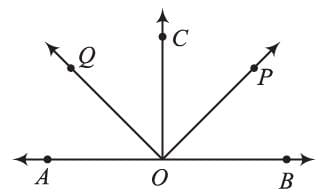Detailed Solution for Math Olympiad Test: Lines and Angles- 1 - Question 3

∵ AOB is a straight line
∴ ∠AOB = 180°
∠AOC + ∠BOC = 180°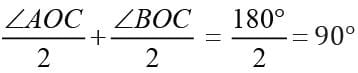⇒ ∠COQ +∠POC = 90°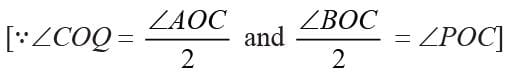⇒ ∠POQ = 90°

Math Olympiad Test: Lines and Angles- 1 - Question 4

If y - x = 10°, then y =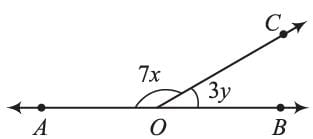Detailed Solution for Math Olympiad Test: Lines and Angles- 1 - Question 4

∠AOC +∠ BOC = 180°
⇒ 7x + 3y = 180° ...(i), and
y - x = 10°
⇒ x = y - 10° ...(ii)
Using (ii) and (i)
7(y - 10°) + 3y = 180°
⇒ 10y = 250°
⇒ y = 25°

Math Olympiad Test: Lines and Angles- 1 - Question 5

POQ is a line. Ray OR is perpendicular to line PQ. OS is another ray lying between rays OP and OR, then ∠POS is equal to: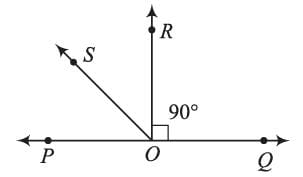Detailed Solution for Math Olympiad Test: Lines and Angles- 1 - Question 5

Let the measure of ∠POS be x°
∵ ∠ POQ = 180°
⇒ ∠POS + ∠ROS + ∠QOR = 180°
⇒ ∠POS + ∠ROS + 90° = 180°
⇒ ∠ROS = (90° - x).
⇒ ∠QOS = 90° + (90° - x) = 180° - x
∴ ∠POS = x = ∠QOS - 2∠ROS.

Math Olympiad Test: Lines and Angles- 1 - Question 6

If q/p = 5, r/p = 3, then r + p =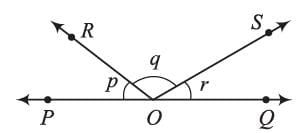Detailed Solution for Math Olympiad Test: Lines and Angles- 1 - Question 6

q = 5p, r = 3p and
∵ ∠POQ = 180°
⇒ p + q + r =  180°
⇒ p + 5p + 3p = 180°
⇒ 9p = 180° ⇒ p = 20°
r = 3p = 3 × 20° = 60°
∴ r + p = 60° + 20° = 80°

Math Olympiad Test: Lines and Angles- 1 - Question 7

In the adjoining figure, ∠AOQ : ∠AOP = 5 : 7, then the measure of ∠BOQ is: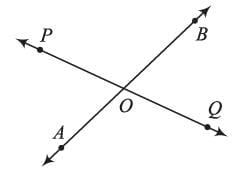Detailed Solution for Math Olympiad Test: Lines and Angles- 1 - Question 7

∵ ∠POQ = 180° [PQ is a straight line]
⇒ ∠AOQ + ∠AOP = 180°
⇒ 5k + 7k = 180°
⇒ 12k = 180°
⇒ k = 15°
∴ ∠BOQ = ∠AOP = 7 × 15° = 105°
[vertically opposite ∠s]

Math Olympiad Test: Lines and Angles- 1 - Question 8

In the adjoining figure, ∠AOC + ∠BOE = 70° and ∠BOD = 40°, then measure of reflex ∠BOE is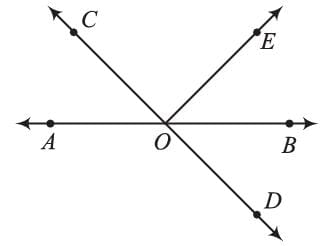Detailed Solution for Math Olympiad Test: Lines and Angles- 1 - Question 8

∠BOD = ∠AOC = 40°
[vertically opposite ∠s]
∵ ∠SOB is a straight angle
∴ ∠AOB = 180°
⇒ ∠AOC + ∠COE + ∠BOE = 180°
⇒ ∠COE = 180° - (∠AOC + ∠BOC)
= 180° - 70° = 110°
⇒ ∠AOC + ∠BOE = 70°
∠AOE = 70° - 40° = 30°
∴ reflex (∠BOC) = 360° - 30 = 330°

Math Olympiad Test: Lines and Angles- 1 - Question 9

Find the value of x - y + z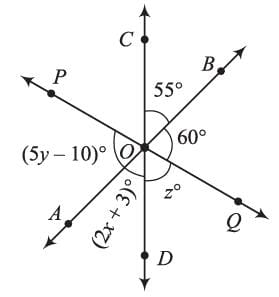Detailed Solution for Math Olympiad Test: Lines and Angles- 1 - Question 9

∵ ∠COD = 180°
⇒ ∠BOC + ∠BOQ + ∠DOQ = 180°
⇒ 55° + 60° + z = 180°
⇒ z = 65°
Similarly ∠BOC = ∠AOD
⇒ 2x + 3° = 55°
⇒ x = 26°
and,
∠AOP = ∠BOQ  [vertically opposite ∠s]
⇒ 5y - 10° = 60°
⇒ y = 70°/5 = 14°
∴ x - y + z = 26° - 14° + 65° = 77°

Math Olympiad Test: Lines and Angles- 1 - Question 10

In the adjoining figure, AB || CD and, PQ ⊥ AB, find the measure of ∠PCM.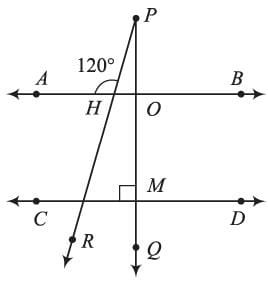Detailed Solution for Math Olympiad Test: Lines and Angles- 1 - Question 10

∠ANP + ∠PNO = 180° [Straight angle]
⇒ ∠PNO = 180° - 120° = 60°
∴ ∠PNO = ∠PCM = 60° [corresponding ∠S]

## Mathematics Olympiad for Class 9

1 videos|43 tests
 Use Code STAYHOME200 and get INR 200 additional OFF Use Coupon Code
Information about Math Olympiad Test: Lines and Angles- 1 Page
In this test you can find the Exam questions for Math Olympiad Test: Lines and Angles- 1 solved & explained in the simplest way possible. Besides giving Questions and answers for Math Olympiad Test: Lines and Angles- 1, EduRev gives you an ample number of Online tests for practice

## Mathematics Olympiad for Class 9

1 videos|43 tests